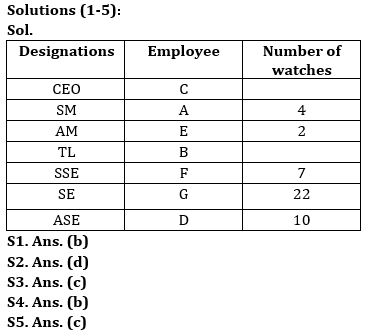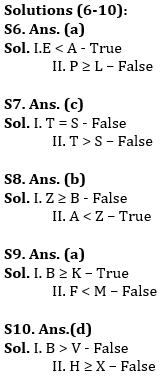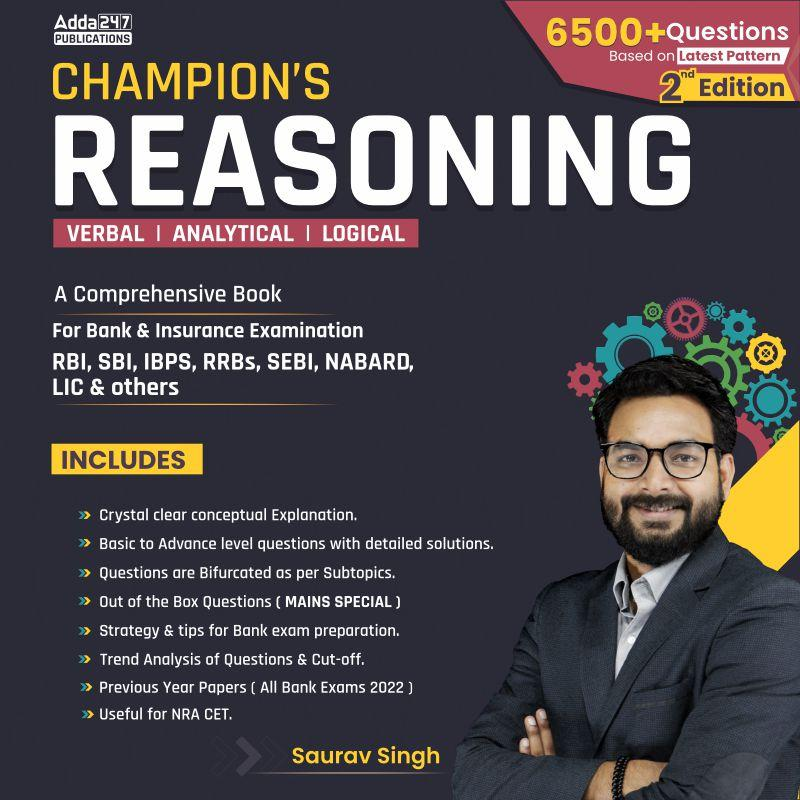Latest Banking jobs   »   Reasoning Quiz For IBPS PO Prelims...

# Reasoning Quiz For IBPS PO Prelims 2023-04th September

Directions (1-5): Answer the questions based on the information given below.
Seven employees (A, B, C, D, E, F and G) of a company have different designations among- CEO, SM, AM, TL, SSE, SE and ASE, such that CEO is the senior most designation and ASE is the junior most designation. Five employees have different number (2, 4, 7, 10 and 22) of watches with them.
E who is AM, has 2 watches less than A. A is four designations senior to G. D who has 3 watches more than F, is junior than G. C is three designations senior to B. SM does not have odd number of watches. Difference between the number of watches of SE and ASE is more than 6.

Q1. Who is the CEO?
(a) A
(b) C
(c) F
(d) G
(e) None of these

Q2. How many persons are senior than F?
(a) One
(b) Two
(c) Three
(d) Four
(e) None of these

Q3. What is the sum of the number of watches of SM and SE?
(a) 14
(b) 24
(c) 26
(d) 29
(e) None of these

Q4. How many persons are senior than B?
(a) Four
(b) Three
(c) Five
(d) Six
(e) None of these

Q5. F and G are ___ and _____ respectively.
(a) SM, SSE
(b) AM, SE
(c) SSE, SE
(d) SE, ASE
(e) None of these

Directions (6-10): In the following questions, a statement(s) is given, followed by some conclusions. Study the following statements carefully and answer the questions accordingly.

Q6. Statements: P > D ≥ E = T ≤ S < L ≤ A
Conclusions: I.E < A
II.P ≥ L
(a) If only conclusion I follows
(b) If only conclusion II follows
(c) If either conclusion I or II follows
(d) If neither conclusion I nor II follows
(e) If both conclusions I and II follow

Q7. Statements: E = P > C ≥ D = S ≤ R < L ≤ A, T ≥ C > Z = N
Conclusions: I. T = S
II. T > S
(a) If only conclusion I follows
(b) If only conclusion II follows
(c) If either conclusion I or II follows
(d) If neither conclusion I nor II follows
(e) If both conclusions I and II follow

Q8. Statements: B = M < Q ≤ Z ≥ S = T, Q = I > A
Conclusions: I. Z ≥ B
II. A < Z
(a) If only conclusion I follows
(b) If only conclusion II follows
(c) If either conclusion I or II follows
(d) If neither conclusion I nor II follows
(e) If both conclusions I and II follow

Q9. Statements: Z > T ≥ F, B ≥ I ≥ J = K > Z > M > N
Conclusions: I. B ≥ K
II. F < M
(a) If only conclusion I follows
(b) If only conclusion II follows
(c) If either conclusion I or II follows
(d) If neither conclusion I nor II follows
(e) If both conclusions I and II follows

Q10. Statements: B = H ≥ V < W > X > Y > Z
Conclusions: I. B > V
II. H ≥ X
(a) If only conclusion I follows
(b) If only conclusion II follows
(c) If either conclusion I or II follows
(d) If neither conclusion I nor II follows
(e) If both conclusions I and II follows

Solutions## FAQs

### When will the IBPS PO prelims 2023 be conducted?

IBPS PO Prelims will be conducted on 23, 30 September, and 1 October 2023.

#### Congratulations!Union Budget 2023-24: Free PDF